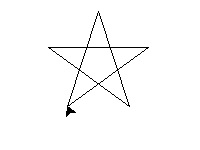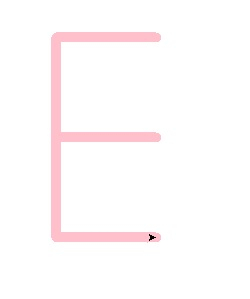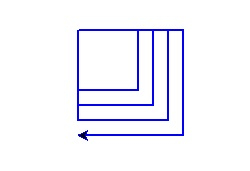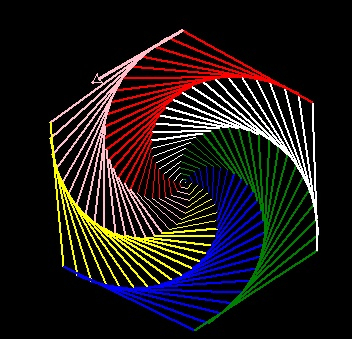# Turtle graphics using Python

PythonServer Side ProgrammingProgramming

Turtle is a Python library to draw graphics. After we import Turtle we can give commands like forward, backward, right, left etc. This commands will draw different shapes when we. When We combine Search commands we can create many nice graphics in the below example we will see some simple scenarios and then some Complex ones where nice graphics is created.

## Simple Turtle Commands

• forward(10)  It moves the turtle (arrow) forward by 10 pixels.

• backward(5)  It moves the turtle (arrow) backward by 5 pixels

•  right(35)  It moves the turtle (arrow) clockwise by an angle of 35 degrees.

• left(55)  It moves the turtle (arrow) counter-clockwise by an angle of 55 degrees

•  goto(x,y)  It moves the turtle (arrow) to the position x, y

•  dot() It creates a dot in the current position.

•  shape(‘circle’) It draws a circle shape.

## Examples

Let’s see some drawings using some simple commands.

### Draw a Star

In the below program we draw a start. We choose appropriate steps to move the cursor forward and then right continuously to get this result.

## Example

import turtle
star = turtle.Turtle()
for i in range(100):
star.forward(100)
star.right(144)
turtle.done()

Running the above code gives us the following result

## Output### Draw Letter E

We follow a similar approach where the turtle moves in all four directions to create the English alphabet E.

## Example

import turtle
t=turtle.Turtle()
t.penup()
t.setpos(-20,40)
t.pendown()
t.pensize(10)
t.pencolor("pink")
t.forward(100)
t.backward(100)
t.right(90)
t.forward(100)
t.left(90)
t.forward(100)
t.backward(100)
t.right(90)
t.forward(100)
t.left(90)
t.forward(100)
turtle.done()

Running the above code gives us the following result

## Output### Multiple Squares

In the next example we see the drawing of multiple squares all starting from a common point. We sue the usual simple commands to go forward, backward and then turn 90 degrees.

## Example

import turtle
mult_square=turtle.Turtle()
def Multiple_Squares(length, colour):
mult_square.pencolor(colour)
mult_square.pensize(2)
mult_square.forward(length)
mult_square.right(90)
mult_square.forward(length)
mult_square.right(90)
mult_square.forward(length)
mult_square.right(90)
mult_square.forward(length)
mult_square.right(90)
for i in range(60,120,15):
Multiple_Squares(i,"blue")
turtle.done

Running the above code gives us the following result

## Output### A spiral hexagon

This is a very interesting example where we use turtle to create a spiral structure. The final shape is a hexagon and there are various colours used in producing the sides of the hexagon.

## Example

import turtle
colors = [ "pink","yellow","blue","green","white","red"]
sketch = turtle.Pen()
turtle.bgcolor("black")
for i in range(200):
sketch.pencolor(colors[i % 6])
sketch.width(i/100 + 1)
sketch.forward(i)
sketch.left(59)

Running the above code gives us the following result